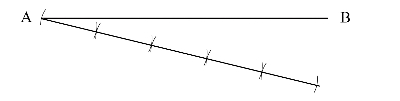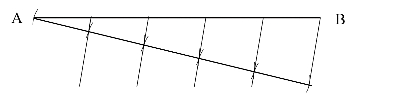## Dividing a Line Equally without a Ruler

Anyone who has picked up a pencil and wanted to make an accurate drawing knows the most important thing is proportions. That means you need to divide things up. Sometimes into several pieces. Now you can eyeball it but if you want to get to a better level of accuracy then you want to use a few simple techniques that will take your drawing to the next level.

Dividing a Line Segment into 5 Equal Parts

Now I can use this method to divide it into any number of parts but for this exercise it will divide it into five.

1. First draw the line you need to divide using a straight edge.2. Draw a line of “any angle”(it doesn’t matter the angle)  from point “A”. As pictured below.3. Using a compass or a divider or even a ruler or even two random marks of any length. Mark off five equal spaces along your line.4. Connect you last marked space to point “B.”(This is very important)  Then using parallel lines connect the marked points to the line “AB”.5. Now you have a line that is divided equally into 5 parts.Now the great part is you can do this by hand the same process and you will divide things much more accurately and quickly than you ever thought possible. Give it a try.

Also this is done with straight edges and such but do this by hand will give you much more accurate divisions even though your lines won’t be as straight. You will be far more accurate than if you “Eye Ball it”.

Where this really becomes important is this can be used in perspective as well. That’s where the true power of this shines. It a variation but the process is essentially the same.

To Find Other Drawing Insights click Drawing Tips

This site uses Akismet to reduce spam. Learn how your comment data is processed.